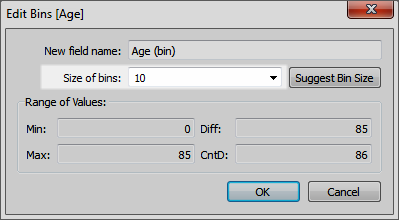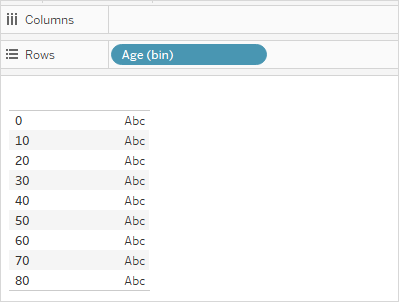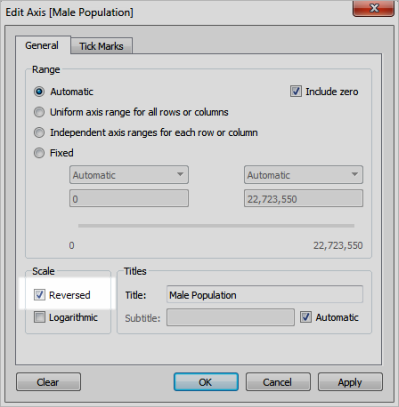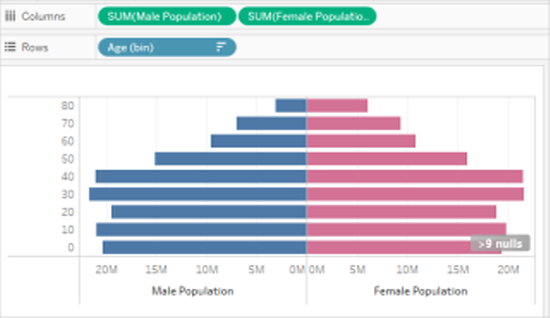# Create a Population Pyramid

A population pyramid, also known as an age structure diagram, shows the distribution of various age groups in a population.

A common distribution often used with this type of visualization is female and male populations by age. To create a population pyramid using Tableau, first separate the population (measure) into two groups, females and males, and then create "bins" for the age cohorts you want to represent in the population pyramid.

For example, suppose you are working with a table from the United States Census Bureau that contains population, gender, and age data.

To create a bin and divide a measure into two groups.

1. Click Download Workbook in the upper-right corner and then open the workbook.

2. Select Worksheet > Clear > Sheet.

3. In the Data pane, right-click the Age field and select Create > Bins.

4. In the Create Bins dialog box, enter a bin size based on the age groups you're interested in focusing on, and then click OK.

In this example, the bin size is 10. This means that the age cohorts are defined by increments of 10 years.5. Drag the bin you just created to the Rows shelf.6. Select Analysis > Create a Calculated Field, and then do the following:

• Enter a name for the calculation. For this example, enter Male Population.

• Enter a formula similar to the following to isolate the male constituent from population:

`IF [Gender] = 1 THEN [ESTBASE2010] END`

In this case, the census data has defined the Gender value for male as "1." The field "ESTBASE2010" contains estimated population values.

7. Similar to the step 5, select Analysis > Create a Calculated Field, and then do the following:

• Enter a name for the calculation. For this example, enter Female Population.

• Enter a formula similar to the following to isolate the female constituent from population:

`IF [Gender] = 2 THEN [ESTBASE2010] END`

In this case, the census data has defined the Gender value for female as "2." The field "ESTBASE2010" contains estimated population values.

8. Drag the calculated fields you created to the Columns shelf and the Gender field to the Color shelf.

Note: To change the color assignments, click the Color shelf and then click Edit Colors.

9. Right-click the axis for Male Population, select Edit Axis, and then select the check box for Reversed to reverse the order the values display on the axis, and then click OK.After changing the sort order of the bin, the population pyramid looks like the following: